Tamil Nadu Board of Secondary EducationHSC Science Class 11

# Tamil Nadu Board Samacheer Kalvi solutions for Class 11th Physics Volume 1 and 2 Answers Guide chapter 11 - Waves [Latest edition]

#### Chapters## Solutions for Chapter 11: Waves

Below listed, you can find solutions for Chapter 11 of Tamil Nadu Board of Secondary Education Tamil Nadu Board Samacheer Kalvi for Class 11th Physics Volume 1 and 2 Answers Guide.

Evaluation
Evaluation [Pages 275 - 279]

### Tamil Nadu Board Samacheer Kalvi solutions for Class 11th Physics Volume 1 and 2 Answers Guide Chapter 11 Waves Evaluation [Pages 275 - 279]

#### Multiple choice questions

Evaluation | Q I. 1. | Page 275

A student tunes his guitar by striking a 120 Hertz with a tuning fork, and simultaneously plays the 4th string on his guitar. By keen observation, he hears the amplitude of the combined sound oscillating thrice per second. Which of the following frequencies is the most likely the frequency of the 4th string on his guitar?

• 130

• 117

• 110

• 120

Evaluation | Q I. 2. | Page 275

A transverse wave moves from a medium A to a medium B. In medium A, the velocity of the transverse wave is 500 ms-1 and the wavelength is 5 m. The frequency and the wavelength of the wave in medium B when its velocity is 600 ms-1, respectively are:

• 120 Hz and 5 m

• 100 Hz and 5 m

• 120 Hz and 6 m

• 100 Hz and 6 m

Evaluation | Q I. 3. | Page 275

For a particular tube, among six harmonic frequencies below 1000 Hz, only four harmonic frequencies are given: 300 Hz, 600 Hz, 750 Hz and 900 Hz. What are the two other frequencies missing from this list?

• 100 Hz, 150 Hz

• 150 Hz, 450 Hz

• 450 Hz, 700 Hz

• 700 Hz, 800 Hz

Evaluation | Q I. 4. | Page 275

Which of the following options is correct?

 A B (1) Quality (A) Intensity (2) Pitch (B) Waveform (3) Loudness (C) Frequency

Options for (1), (2) and (3), respectively are

• (B), (C) and (A)

• (C), (A) and (B)

• (A), (B) and (C)

• (B), (A) and (C)

Evaluation | Q I. 5. | Page 275

Compare the velocities of the wave forms given below, and choose the correct option.

1.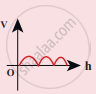2.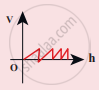3.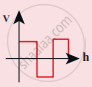4.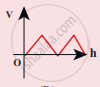where, vA, vB, vC and vD are velocities given in (a), (b), (c) and (d), respectively.

• vA > vB > vD > vC

• vA < vB < vD < vC

• vA = vB = vD = vC

• vA > vB = vD > vC

Evaluation | Q I. 6. | Page 275

A sound wave whose frequency is 5000 Hz travels in air and then hits the water surface. The ratio of its wavelengths in water and air is ______.

• 4.30

• 0.23

• 5.30

• 1.23

Evaluation | Q I. 7. | Page 275

A person standing between two parallel hills fires a gun and hears the first echo after t1 sec and the second echo after t2 sec. The distance between the two hills is:

• ("v"("t"_1 - "t"_2))/2

• ("v"("t"_1"t"_2))/(2("t"_1 + "t"_2))

• v(t1 + t2)

• ("v"("t"_1 + "t"_2))/2

Evaluation | Q I. 8. | Page 276

An air column in a pipe which is closed at one end, will be in resonance with the vibrating body of frequency 83Hz. Then the length of the air column is ______.

• 1.5 m

• 0.5 m

• 1.0 m

• 2.0 m

Evaluation | Q I. 9. | Page 276

The displacement y of a wave travelling in the x direction is given by y = (2 x 10-3) sin (300 "t" -  2x  +  pi/4), where x and y are measured in metres and t in second. The speed of the wave is:

• 150 ms-1

• 300 ms-1

• 450 ms-1

• 600 ms-1

Evaluation | Q I. 10. | Page 276

Consider two uniform wires vibrating simultaneously in their fundamental notes. The tensions, densities, lengths and diameter of the two wires are in the ratio 8 : 1, 1 : 2, x : y and 4 : 1 respectively. If the note of the higher pitch has a frequency of 360 Hz and the number of beats produced per second is 10, then the value of x : y is

• 36 : 35

• 35 : 36

• 1 : 1

• 1 : 2

Evaluation | Q I. 11. | Page 276

Which of the following represents a wave.

• (x – vt)³

• x(x + vt)

• 1/(x + "vt")

• sin(x + vt)

Evaluation | Q I. 12. | Page 276

A man sitting on a swing which is moving to an angle of 60° from the vertical is blowing a whistle which has a frequency of 2.0 k Hz. The whistle is 2.0 m from the fixed support point of the swing. A sound detector which detects the whistle sound is kept in front of the swing. The maximum frequency the sound detector detected is

• 2.027 kHz

• 1.914 kHz

• 9.14 kHz

• 1.011 kHz

Evaluation | Q I. 13. | Page 276

Let y = 1/(1 + x^2) at  t = 0 be the amplitude of the wave propagating in the positive x-direction. At t = 2s, the amplitude of the wave propagating becomes y = 1/(1 + (x - 2)^2). Assume that the shape of the wave does not
change during propagation. The velocity of the wave is:

• 0.5 ms-1

• 1.0 ms-1

• 1.5 ms-1

• 2.0 ms-1

Evaluation | Q I. 14. | Page 276

A uniform rope having mass m hangs vertically from a rigid support. A transverse wave pulse is produced at the lower end. Which of the following plots shows the correct variation of speed v with height h from the lower end?

•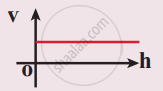•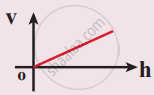•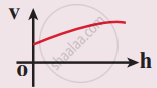•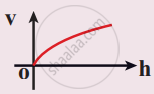Evaluation | Q I. 15. | Page 277

An organ pipe A closed at one end is allowed to vibrate in its first harmonic and another pipe B open at both ends is allowed to vibrate in its third harmonic. Both A and B are in resonance with a given tuning fork. The ratio of the length of A and B is

• $\frac{8}{3}$

• $\frac{3}{8}$

• $\frac{1}{6}$

• $\frac{1}{3}$

Evaluation | Q II. 1. | Page 277

What is meant by waves?

Evaluation | Q II. 2. | Page 277

Write down the types of waves.

Evaluation | Q II. 3. | Page 277

What are transverse waves? Give one example.

Evaluation | Q II. 4. | Page 277

What are longitudinal waves? Give one example.

Evaluation | Q II. 5. | Page 277

Define wavelength.

Evaluation | Q II. 6. | Page 277

Write down the relation between frequency, wavelength and velocity of a wave.

Evaluation | Q II. 7. | Page 277

What is meant by interference of waves?

Evaluation | Q II. 8. | Page 277

Explain the beat phenomenon.

Evaluation | Q II. 9. a. | Page 277

Define intensity of sound.

Evaluation | Q II. 9. b. | Page 277

Define loudness of sound.

Evaluation | Q II. 10. | Page 277

What is Doppler effect?

Evaluation | Q II. 11. | Page 277

Explain red shift and blue shift in Doppler Effect.

Evaluation | Q II. 12. | Page 277

What is meant by end correction in resonance air column apparatus?

Evaluation | Q II. 13. | Page 277

Sketch the function y = x + a. Explain your sketch.

Evaluation | Q II. 14. | Page 277

Write down the factors affecting velocity of sound in gases.

Evaluation | Q II. 15. | Page 277

What is meant by an echo? Explain.

Evaluation | Q III. 1. | Page 277

Discuss how ripples are formed in still water.

Evaluation | Q III. 2. | Page 277

Briefly explain the difference between traveling waves and standing waves.

Evaluation | Q III. 3. | Page 277

Show that the velocity of a traveling wave produced in a string is v = sqrt("T"/mu).

Evaluation | Q III. 4. | Page 277

Describe Newton’s formula for velocity of sound waves in air and also discuss Laplace’s correction.

Evaluation | Q III. 5. | Page 277

Write short notes on reflection of sound waves from plane and curved surfaces.

Evaluation | Q III. 6. | Page 277

Briefly explain the concept of the superposition principle.

Evaluation | Q III. 7. | Page 277

Explain how the interference of waves is formed.

Evaluation | Q III. 8. | Page 277

Describe the formation of beats.

Evaluation | Q III. 9. a. | Page 277

What is a stationary wave?

Evaluation | Q III. 9. b. | Page 277

Explain the formation of stationary waves.

Evaluation | Q III. 9. c. | Page 277

Write the characteristics of stationary waves.

Evaluation | Q III. 10. | Page 277

Discuss the law of transverse vibrations in stretched strings.

Evaluation | Q III. 11. | Page 277

Explain the concepts of fundamental frequency, harmonics and overtones in detail.

Evaluation | Q III. 12. | Page 277

What is a sonometer? Give its construction and working. Explain how to determine the frequency of tuning fork using a sonometer.

Evaluation | Q III. 13. a. | Page 278

Write a short note on intensity.

Evaluation | Q III. 13. b. | Page 278

Write a short note on loudness.

Evaluation | Q III. 14. (a) | Page 278

Explain how overtone is produced in a closed organ pipe.

Evaluation | Q III. 14. (b) | Page 278

Explain how overtone is produced in a Open organ pipe.

Evaluation | Q III. 15. | Page 278

How will you determine the velocity of sound using resonance air column apparatus?

Evaluation | Q III. 16. (1) | Page 278

Discuss the following case:

Source in motion and Observer at rest

1. Source moves towards observer
2. Source moves away from the observer
Evaluation | Q III. 16 a. | Page 278

What is meant by the Doppler effect?

Evaluation | Q III. 16. (2) | Page 278

Discuss the following case-

Observer in motion and Source at rest.

1. Observer moves towards Source
2. Observer resides away from the Source
Evaluation | Q III. 16. (3) | Page 278

Discuss the following case-

Both are in motion

1. Source and Observer approach each other
2. Source and Observer resides from each other
3. Source chases Observer
4. Observer chases Source

#### Numerical Problems

Evaluation | Q IV. 1. | Page 278

The speed of a wave in a certain medium is 900 m/s. If 3000 waves passes over a certain point of the medium in 2 minutes, then compute its wavelength?

Evaluation | Q IV. 2. | Page 278

Consider a mixture of 2 mol of helium and 4 mol of oxygen. Compute the speed of sound in this gas mixture at 300 K.

Evaluation | Q IV. 3. | Page 278

A ship in a sea sends SONAR waves straight down into the seawater from the bottom of the ship. The signal reflects from the deep bottom bedrock and returns to the ship after 3.5 s. After the ship moves to 100 km it sends another signal which returns back after 2 s. Calculate the depth of the sea in each case and also compute the difference in height between two cases.

Evaluation | Q IV. 4. | Page 278

A sound wave is transmitted into a tube as shown in the figure. The sound wave splits into two waves at point A which recombine at point B. Let R be the radius of the semi-circle which is varied until the first minimum. Calculate the radius of the semi-circle if the wavelength of the sound is 50.0 m.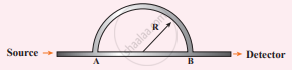Evaluation | Q IV. 5. | Page 278

N tuning forks are arranged in order of increasing frequency and any two successive tuning forks give n beats per second when sounded together. If the last fork gives double the frequency of the first (called as octave), Show that the frequency of the first tuning fork is f = (N – 1)n.

Evaluation | Q IV. 6. | Page 278

Let the source propagate a sound wave whose intensity at a point (initially) be I. Suppose we consider a case when the amplitude of the sound wave is doubled and the frequency is reduced to one-fourth. Calculate now the new intensity of sound at the same point?

Evaluation | Q IV. 7. | Page 279

Consider two organ pipes of the same length in which one organ pipe is closed and another organ pipe is open. If the fundamental frequency of closed pipe is 250 Hz. Calculate the fundamental frequency of the open pipe.

Evaluation | Q IV. 8. | Page 279

Police in a siren car moving with a velocity 20 ms chases a thief who is moving in a car with a velocity v0 ms-1. The police car sounds at frequency 300 Hz, and both of them move towards a stationary siren of frequency 400 Hz. Calculate the speed in which thief is moving. (Assume the thief does not observe any beat)

Evaluation | Q IV. 9. | Page 279

Consider the following function-

1. y = x² + 2 α tx
2. y = (x + vt)²

which among the above function can be characterized as a wave?

#### Conceptual Questions

Evaluation | Q V. 1. | Page 279

Why is it that transverse waves cannot be produced in a gas? Can the transverse waves be produced in solids and liquids?

Evaluation | Q V. 2. | Page 279

Why is the roar of our national animal different from the sound of a mosquito?

Evaluation | Q V. 3. | Page 279

A sound source and listener are both stationary and a strong wind is blowing. Is there a Doppler effect?

Evaluation | Q V. 4. | Page 279

In an empty room why is it that a tone sounds louder than in the room having things like furniture etc.

Evaluation | Q V. 5. | Page 279

How do animals sense impending danger of hurricane?

Evaluation | Q V. 6. | Page 279

Is it possible to realize whether a vessel kept under the tap is about to fill with water?

## Solutions for Chapter 11: Waves

Evaluation## Tamil Nadu Board Samacheer Kalvi solutions for Class 11th Physics Volume 1 and 2 Answers Guide chapter 11 - Waves

Shaalaa.com has the Tamil Nadu Board of Secondary Education Mathematics Class 11th Physics Volume 1 and 2 Answers Guide Tamil Nadu Board of Secondary Education solutions in a manner that help students grasp basic concepts better and faster. The detailed, step-by-step solutions will help you understand the concepts better and clarify any confusion. Tamil Nadu Board Samacheer Kalvi solutions for Mathematics Class 11th Physics Volume 1 and 2 Answers Guide Tamil Nadu Board of Secondary Education 11 (Waves) include all questions with answers and detailed explanations. This will clear students' doubts about questions and improve their application skills while preparing for board exams.

Further, we at Shaalaa.com provide such solutions so students can prepare for written exams. Tamil Nadu Board Samacheer Kalvi textbook solutions can be a core help for self-study and provide excellent self-help guidance for students.

Concepts covered in Class 11th Physics Volume 1 and 2 Answers Guide chapter 11 Waves are Reflection of Sound, Introduction to Waves, Terms and Definitions Used in Wave Motion, Velocity of Waves in Different Media, Propagation of Sound Waves, Progressive Wave Or Travelling Waves, Superposition Principle, Standing Waves, Intensity and Loudness, Vibration of Air Column, Doppler Effect.

Using Tamil Nadu Board Samacheer Kalvi Class 11th Physics Volume 1 and 2 Answers Guide solutions Waves exercise by students is an easy way to prepare for the exams, as they involve solutions arranged chapter-wise and also page-wise. The questions involved in Tamil Nadu Board Samacheer Kalvi Solutions are essential questions that can be asked in the final exam. Maximum Tamil Nadu Board of Secondary Education Class 11th Physics Volume 1 and 2 Answers Guide students prefer Tamil Nadu Board Samacheer Kalvi Textbook Solutions to score more in exams.

Get the free view of Chapter 11, Waves Class 11th Physics Volume 1 and 2 Answers Guide additional questions for Mathematics Class 11th Physics Volume 1 and 2 Answers Guide Tamil Nadu Board of Secondary Education, and you can use Shaalaa.com to keep it handy for your exam preparation.